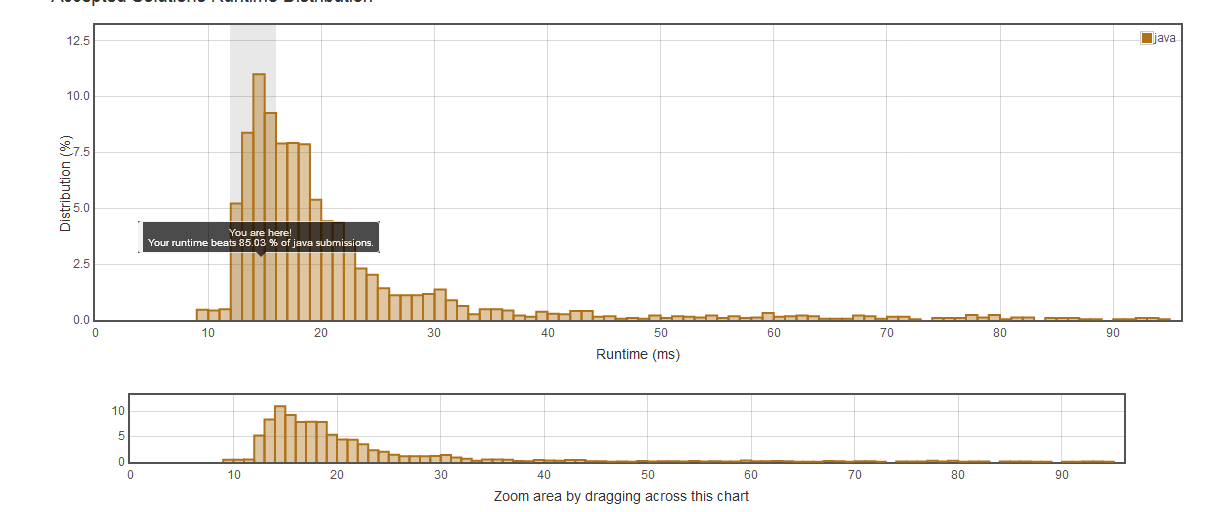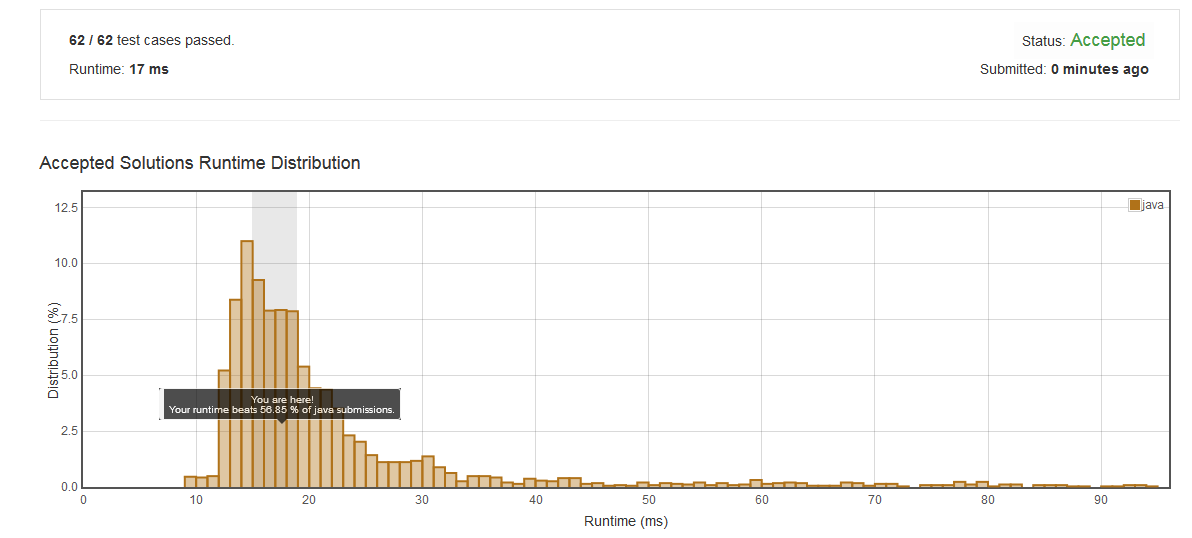# LeetCode 657. Judge Route Circle

problem:

 Initially, there is a Robot at position (0, 0). Given a sequence of its moves, judge if this robot makes a circle, which means it moves back to the original place.

The move sequence is represented by a string. And each move is represent by a character. The valid robot moves are R (Right), L (Left), U (Up) and D (down). The output should be true or false representing whether the robot makes a circle.

Example 1:

Input: "UD"
Output: true

Example 2:

Input: "LL"
Output: false

first:

import java.util.Stack;class Solution {    public boolean judgeCircle(String moves) {        Stack<Character> stack = new Stack<Character>();        for(int i=0;i<moves.length();i++){            if(stack.size()==0){                stack.push(moves.charAt(i));            } else if(stack.peek()=='U'&&moves.charAt(i)=='D'||                      stack.peek()=='D'&&moves.charAt(i)=='U'||                      stack.peek()=='L'&&moves.charAt(i)=='R'||                      stack.peek()=='R'&&moves.charAt(i)=='L'){                stack.pop();            } else {                stack.push(moves.charAt(i));            }        }                return stack.empty() ? true : false;    }}

result:

Input: "RLUURDDDLU"
Output: false
Expected: true

2:

import java.util.Stack;

class Solution {
public boolean judgeCircle(String moves) {
int rCounts=0, lCounts=0,dCounts=0,uCounts=0;
for(int i=0;i<moves.length();i++){
if(moves.charAt(i)=='U'){
uCounts++;
}
if(moves.charAt(i)=='D'){
dCounts++;
}
if(moves.charAt(i)=='R'){
rCounts++;
}
if(moves.charAt(i)=='L'){
lCounts++;
}
}

return uCounts==dCounts&&rCounts==lCounts ? true : false;
}
}

result:class Solution {
public boolean judgeCircle(String moves) {
int x = 0, y = 0;
for (char move: moves.toCharArray()) {
if (move == 'U') y--;
else if (move == 'D') y++;
else if (move == 'L') x--;
else if (move == 'R') x++;
}
return x == 0 && y == 0;
}
}

result：retry:

class Solution {
public boolean judgeCircle(String moves) {
int rCounts=0, lCounts=0,dCounts=0,uCounts=0;
char[] charArray = moves.toCharArray();
for(int i=0;i<charArray.length;i++){
if(charArray[i]=='U'){
uCounts++;
}
if(charArray[i]=='D'){
dCounts++;
}
if(charArray[i]=='R'){
rCounts++;
}
if(charArray[i]=='L'){
lCounts++;
}
}

return uCounts==dCounts&&rCounts==lCounts ? true : false;
}
}

result:conclusion:

posted @ 2018-04-25 19:05  Zhao_Gang  阅读(78)  评论(0编辑  收藏  举报